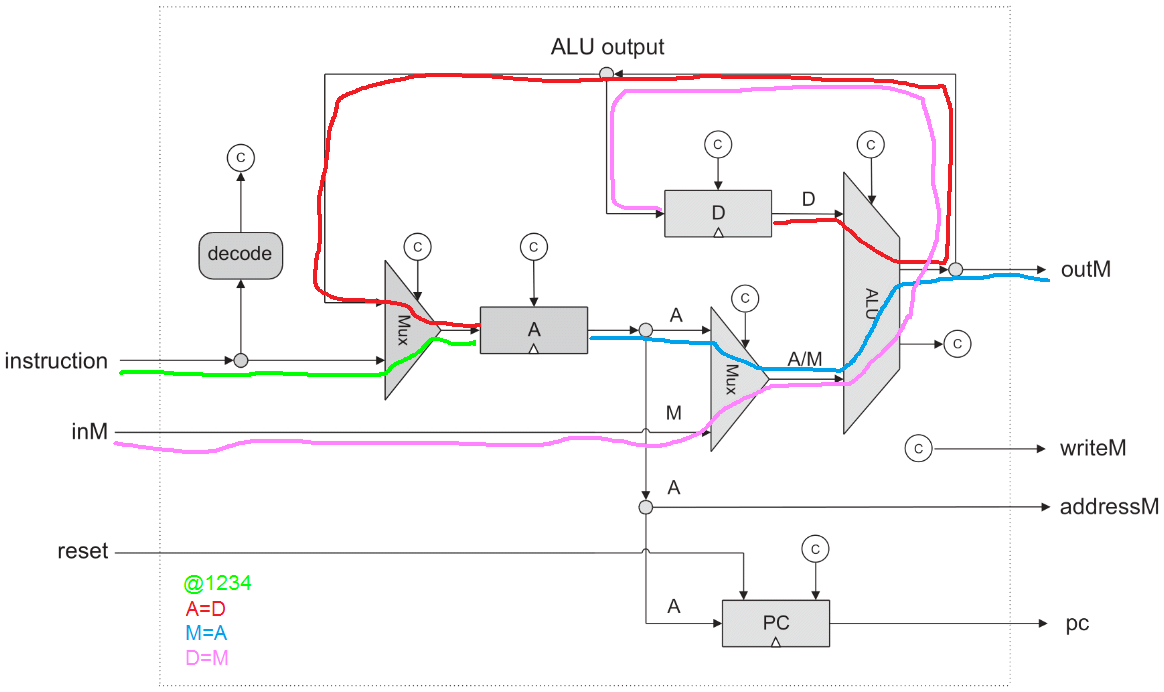# What does the a-bit in a C-instruction mean?ClassicListThreaded11 messagesOpen this post in threaded view
|

## What does the a-bit in a C-instruction mean?

 From the book it says: In the case of a C-instruction, the single a-bit determines whether the ALU will operate on the A register input or on the M input, and the six c-bits determine which function the ALU will compute. I'm looking at the computer nemonic table fig 4.3 and you have one column, a=0, with computations such as D-A , D+A. And another column on the right, a=1, with computations such as D+M, D&M. This has me a little confused because I would've though that if a=1, then the ALU will operate on the A register. But judging from the mnemonic table, it seems like the ALU operates on the A register if a=0. Am I correct in my thinking?
Open this post in threaded view
|

## Re: What does the a-bit in a C-instruction mean?

 'a' doesn't mean 'A' they could have just as easily used 'x' (or any other variable label), as in: x=0 -> use the A register x=1 -> use the M register so yes, when that 4th bit is 0, you'll use the A register, and when its 1, you'll use the M register. edit: technically, its bit
Open this post in threaded view
|

## Re: What does the a-bit in a C-instruction mean?

 Administrator In reply to this post by riverfish Look at the CPU diagram. The ALU 'x' input (first argument) is D and the 'y' input (second argument) is the output of a Mux that selects between A and M. That Mux is what the 'a' bit in C-instructions controls.Open this post in threaded view
|

## Re: What does the a-bit in a C-instruction mean?

 Thanks for the clarification guys. Since you posted the diagram Cadet, I got another unrelated question about the A-register. From what I understand, the A-register either reads the current value in the A register or writes the output of the MUX of Instruction and ALU output into the A register. If that's the case, what would the control bit for the A register represent then? Would it also be the a-bit (i.e. instruction)? Because if a=0, then it means that you want to utilise the A register?
Open this post in threaded view
|

## Re: What does the a-bit in a C-instruction mean?

 Administrator The 'a' bit only has meaning in c-instructions that read from M. It has noting to do with writing to M or writing to the A Register. Remember the Register you wrote in project 3. It has 2 inputs: 'in' and 'load'.  As you wrote, the multiplexor output is connected to the A Register input, so the control signal must be the A Register's 'load'. The D Register's 'load' signal is simple, it needs to be true only during c-instructions that have the "D" dest bit set. The A Register is trickier. Its 'load' needs to be true during c-instructions that have the "A" dest bit set, but 'load' also needs to be true during a-instructions so that the data in the instruction will be loaded into the A Register.
Open this post in threaded view
|

## Re: What does the a-bit in a C-instruction mean?

 So the A register's load bit needs to true when a c-instruction has the "A" dest bit set, i.e. instruction and when the instruction is an A-instruction as well, i.e. instruction? That has me a little confused because I had the Mux before the A register having the control bit as instruction too.
Open this post in threaded view
|

## Re: What does the a-bit in a C-instruction mean?

 Administrator The muxes are like switches that control how data flows through the CPU for various instructions. Here's a quick drawing data paths for some instructions.Open this post in threaded view
|

## Re: What does the a-bit in a C-instruction mean?

Open this post in threaded view
|

## Re: What does the a-bit in a C-instruction mean?

 In reply to this post by cadet1620 I found this reply from another thread: To store a value in the A register, you must use an A instruction, or a C instruction where A is the dest. For example: @42 // stores 42 in the A register A=1 // stores 1 in the A register So the control bit for the A register is an OR between the "A" dest of a C-instruction and the opcode of an A-instruction? As for the Mux that feeds into the A register what would the control bit be?